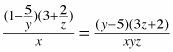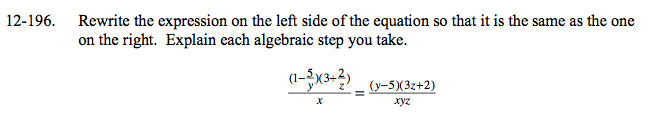### Home > A2C > Chapter 12 > Lesson 12.5.1 > Problem12-196

12-196.
1. Rewrite the expression on the left side of the equation so that it is the same as the one on the right. Explain each algebraic step you take. Homework Help ✎

2.$\left(1-\frac{5}{y}\right)=\left(\frac{y}{y}-\frac{5}{y}\right)=\frac{1}{y}(y-5)$